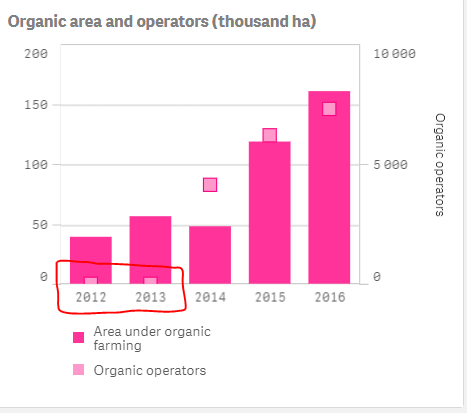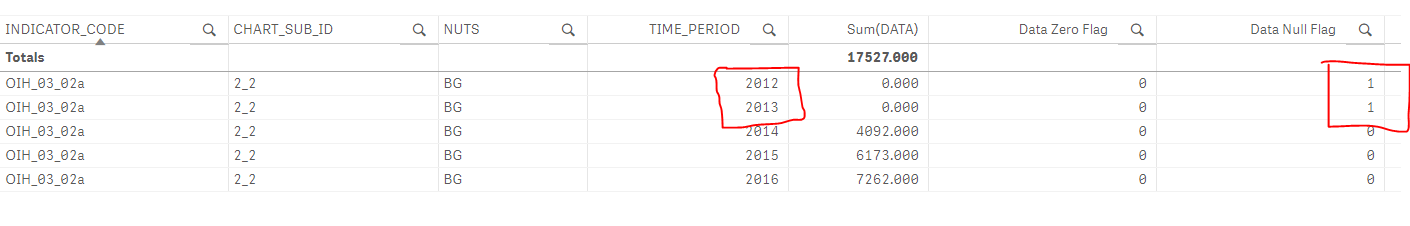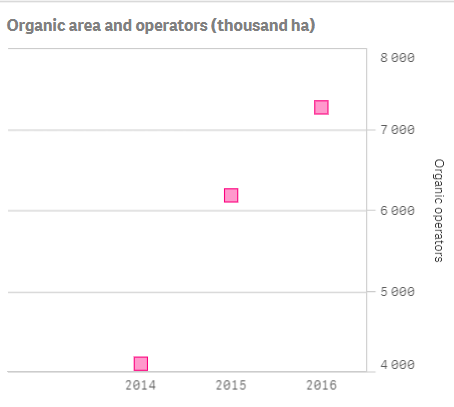# Qlik Sense App Development

Announcements
BARC’s The BI Survey 19 makes it official. BI users love Qlik. GET REPORT
Highlighted
Valued Contributor

## Cannot hide marker when second measure is null/zero

Hi everyone,

I have a combo chart where the value of one measure is null for some years, while the other measure is not null (see 2012 and 2013 below)I have attempted to exclude the nulls from the chart, but this is not working.  The definition is:

if(getSelectedCount([Member State Name])=0,Num(Sum({<CHART_SUB_ID={'2_2'},[Member State Name]= {'European Union'}, TIME_PERIOD={">=2012"},[Data Null Flag]={'0'},[Data Zero Flag]={'0'}>}DATA),'# ##0'),Num(Sum({<CHART_SUB_ID={'2_2'},[Member State Name]= {\$(=vMSValue)}, TIME_PERIOD={">=2012"},[Data Null Flag]={'0'},[Data Zero Flag]={'0'}>}DATA),'# ##0'))

From displaying this data in a table (see below), I can see that for 2012 and 2013 we have NULL values.And if I remove the first measure, the null records are hidden:So, it seems to me that the instruction in the set analysis is simply being ignored when two measures are used in a combo chart. I have also tried simply adding if([Data Zero Flag]='0'...., but this does not work either.

Does anyone have any workaround for this?

Tags (3)
1 Solution

Accepted SolutionsMVP

## Re: Cannot hide marker when second measure is null/zero

In that case, may be only change your second expression to this

If(if(getSelectedCount([Member State Name])=0,

Num(Sum({<CHART_SUB_ID={'2_2'},[Member State Name]= {'European Union'}, TIME_PERIOD={">=2012"},[Data Null Flag]={'0'},[Data Zero Flag]={'0'}>}DATA),'# ##0'),

Num(Sum({<CHART_SUB_ID={'2_2'},[Member State Name]= {\$(=vMSValue)}, TIME_PERIOD={">=2012"},[Data Null Flag]={'0'},[Data Zero Flag]={'0'}>}DATA),'# ##0')) <> 0,

if(getSelectedCount([Member State Name])=0,

Num(Sum({<CHART_SUB_ID={'2_2'},[Member State Name]= {'European Union'}, TIME_PERIOD={">=2012"},[Data Null Flag]={'0'},[Data Zero Flag]={'0'}>}DATA),'# ##0'),

Num(Sum({<CHART_SUB_ID={'2_2'},[Member State Name]= {\$(=vMSValue)}, TIME_PERIOD={">=2012"},[Data Null Flag]={'0'},[Data Zero Flag]={'0'}>}DATA),'# ##0')))6 RepliesMVP

## Re: Cannot hide marker when second measure is null/zero

Would you be able to share a qvf to show the issue?

Valued Contributor

## Re: Cannot hide marker when second measure is null/zero

Hi Sunny,

Thanks for responding.  I have attached a qvf file in which you will be able to see the issue.MVP

## Re: Cannot hide marker when second measure is null/zero

May be try this

If(Column(2) <> 0,

if(getSelectedCount([Member State Name])=0,

Num(Sum({<CHART_SUB_ID={'2_1'},[Member State Name]= {'European Union'}, TIME_PERIOD={">=2012"},[Data Null Flag]={'0'},[Data Zero Flag]={'0'}>}DATA/1000),'# ##0'),

Num(Sum({<CHART_SUB_ID={'2_1'},[Member State Name]= {\$(=vMSValue)}, TIME_PERIOD={">=2012"},[Data Null Flag]={'0'},[Data Zero Flag]={'0'}>}DATA/1000),'# ##0')))Valued Contributor

## Re: Cannot hide marker when second measure is null/zero

Hi, thanks but this hides both measures, whereas I would like to show the first measure but hide the second.MVP

## Re: Cannot hide marker when second measure is null/zero

In that case, may be only change your second expression to this

If(if(getSelectedCount([Member State Name])=0,

Num(Sum({<CHART_SUB_ID={'2_2'},[Member State Name]= {'European Union'}, TIME_PERIOD={">=2012"},[Data Null Flag]={'0'},[Data Zero Flag]={'0'}>}DATA),'# ##0'),

Num(Sum({<CHART_SUB_ID={'2_2'},[Member State Name]= {\$(=vMSValue)}, TIME_PERIOD={">=2012"},[Data Null Flag]={'0'},[Data Zero Flag]={'0'}>}DATA),'# ##0')) <> 0,

if(getSelectedCount([Member State Name])=0,

Num(Sum({<CHART_SUB_ID={'2_2'},[Member State Name]= {'European Union'}, TIME_PERIOD={">=2012"},[Data Null Flag]={'0'},[Data Zero Flag]={'0'}>}DATA),'# ##0'),

Num(Sum({<CHART_SUB_ID={'2_2'},[Member State Name]= {\$(=vMSValue)}, TIME_PERIOD={">=2012"},[Data Null Flag]={'0'},[Data Zero Flag]={'0'}>}DATA),'# ##0')))Valued Contributor

## Re: Cannot hide marker when second measure is null/zero

Hi Sunny,

Thanks, that works perfectly.

Regards,

Richard